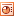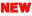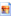# All Other AlgebraExpressions, Identities, Formulae, Equations

A set of common and important expressions, equations, formulae and identities for students to classify and discuss. These are intended to extend and prompt discussion as well as fulfill the basic classifications activity.Graphs, Algebra Number Machines (and input/output tables & words)

Pupils consolidate understanding of straight line graphs by cutting up and then matching each line graph to its equation, sentence, input/output table and number machine.Relationships, PDF here

A number machine, algebra, graph, sentence, sequence and input/output table for students to investigate... and hopefully come to the conclusion that all represent the same relationship in different ways. Suggestions for ways to investigate are on slide 4. To extend this rich open task students could create their own set of cards. Lesson plan. A more structured version (PDF here) for students requiring more support.

A subsequent activity is for students to complete the number machine, table, sentence and graph from the algebra. Prompt cards (PDF here)for this activity with lesson plan.Harder GCSE Algebra Question Match-up Cards

A set of 26 harder GCSE algebra questions on simplifying, rearranging and solving. Pupils match up questions to answers and then use as support, or just for checking, to solve questions. PDF here. Here is set of 'rich questions' to go with the activity. Here are the questions sorted by type (and PDF here).Intro to Algebraic Fractions

Recap questions on numeric fractions and then similar questions involving algebraic fractions. A great gentle introduction into what appears a difficult topic; making difficult things easy! Includes answers. PDF here.Intro to the Difference-of-Two-Squares method

21 intelligent variation questions on difference of two squares. Starting from straightforward until they get really quite tricky. Its just the same method throughout applied to increasingly complicated examples. Includes answers. PDF here.GCSE Algebra Extension Questions

A set of questions taken from the AQA Additional Maths specimen paper. A great GCSE extension or Yr12 introduction. PDF here. Ask pupils to order by difficulty using this template and then attempt all that they can. Lesson plan.How Many Records?

Another mathematical conundrum. Adapted from a rather boring example, this time its DJ's Roni Size & Norman Cook arguing about who has the most records. Can you help them figure it out?! (There's a hint on the second page if you need it).Maths Puzzle

A good deduction based puzzle for pupils of many ages.Maths Puzzle 2

A shakedown of understanding of column addition methods, BIDMAS, substitution, factors, multiples... leading to an SMSC consideration of the relative frequencies of letters used in the english language.

 Smartboard file starterLesson planKLO'sGraph of letter frequencies by frequencyGraph of letter frequencies by letterFind the Code

Find the code from the given clues using algebra or otherwise.Circles Equations

Worksheet to check understanding of association of graph of circle to its equation.Line Graphs

MS Excel program to demonstrate the link between equation, input-output tables & their graphs. This is fantastic for whole class demonstrations including getting pupils to draw on the lines and then using the program to check accuracy.Find the Code

Find the code from the given clues using algebra or otherwise.Indices Rule

How the rules of indices work. Follow them and you can't go wrong!Indices

Evaluate the indices (includes answers) and simplify the indices. PDF here. More indices questions.Proportion

Simple ppt of direct & inverse proportion examples.Gravity & the Inverse Square Law

An applied use of inverse proportion. Good but difficult example!Substitution

Simple substitution questions given the values of a, b and c. More complex examples of common formula follows. Useful for getting pupils used to sustituting values into formulas.Algebra Rectangles

Use the parallel sides property of rectangles to work out the missing expressions. (Thanks Hunneyball!)